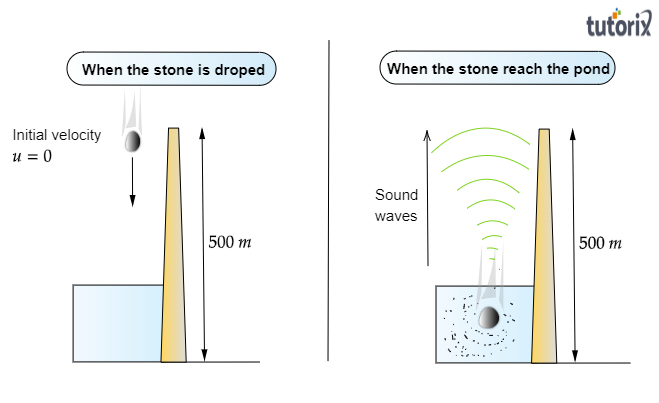# A stone is dropped from the top of a tower $500\ m$ high into a pond of water at the base of the tower. When is the splash heard at the top? Given, $g=10\ ms^{-2}$ and speed of sound $=340\ ms^{-1}$.

Given:
A stone is dropped from the top of a tower $500\ m$ high into a pond of water at the base of the tower.

To do:
To find out when is the splash heard at the top.

Solution:
Height of the tower, $s=500\ m$
Velocity of sound, $v=340\ m/s$
Acceleration due to gravity, $g=10\ m/s^2$

Initial velocity of the stone, $u=0$ $(since\ the\ stone\ is\ initially\ at\ rest)$Time taken by the stone to fall to the base of the tower, $t_1$

According to the second equation of motion:

$S=ut_1+\frac{1}{2}gt_1^2$

$500=0\times t_1+\frac{1}{2}\times10\times t_1^2$

$t_1^2=100$

$t_1=10\ s$

Now, the time taken by the sound to reach the top from the base of the tower is, $t_2=\frac{500}{340}=1.47\ s$

Therefore, the splash is heard at the top after time, $t$

Where, $t=t_1+t_2=10+1.47=11.47\ s$